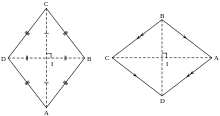# Vector question in 3d

Gold Member

## Homework Statement

The points A,B and C have position vectors , relative to the origin O given by ## OA= i+2j+3k, OB=4j+k , OC=2i+5j-k.## A fourth point D is such that the quadrilateral ABCD is a parallelogram.
i) Find the position vector of D and verify that the parallelogram is a Rhombus.
ii)The plane p is parallel to OA and the line BC lies in p. Find the equation of p,giving your answer in the form ##ax+by+cz=d##

## The Attempt at a Solution

##AB= (4j+k)-(i+2j+3k)=-i+2j-2k, AC= (2i+5j-k)-(i+2j+3k)=i+3j-4k, BC= (2i+5j-k)-(4j+k)=2i+j-2k## i am just groping in the dark here, but i know vectors on same line and parallel vectors should have a scalar or something...relating them

SteamKing
Staff Emeritus
Homework Helper

## Homework Statement

The points A,B and C have position vectors , relative to the origin O given by ## OA= i+2j+3k, OB=4j+k , OC=2i+5j-k.## A fourth point D is such that the quadrilateral ABCD is a parallelogram.
i) Find the position vector of D and verify that the parallelogram is a Rhombus.
ii)The plane p is parallel to OA and the line BC lies in p. Find the equation of p,giving your answer in the form ##ax+by+cz=d##

## The Attempt at a Solution

##AB= (4j+k)-(i+2j+3k)=-i+2j-2k, AC= (2i+5j-k)-(i+2j+3k)=i+3j-4k, BC= (2i+5j-k)-(4j+k)=2i+j-2k## i am just groping in the dark here, but i know vectors on same line and parallel vectors should have a scalar or something...relating them
Let's take things one at a time.

What do you know about the sides of a general quadrilateral figure which would make it a rhombus?

https://en.wikipedia.org/wiki/Rhombus

Hint: it's OK to make a sketch, if that helps.

Gold Member
i am unable to make a sketch on this application, ok for a quadrilateral the opposite sides are equal in terms of distance ##d##
In Euclidean geometry, a rhombus(◊), plural rhombi or rhombuses, is a simple (non-self-intersecting) quadrilateral whose four sides all have the same length. Another name is equilateral quadrilateral, since equilateral means that all of its sides are equal in length. The rhombus is often called a diamond, after the diamonds suit in playing cards which resembles the projection of an octahedral diamond, or a lozenge, though the former sometimes refers specifically to a rhombus with a 60° angle (see Polyiamond), and the latter sometimes refers specifically to a rhombus with a 45° angle.

Every rhombus is a parallelogram and a kite. A rhombus with right angles is a square.SteamKing
Staff Emeritus
Homework Helper
i am unable to make a sketch on this application, ok for a quadrilateral the opposite sides are equal in terms of distance ##d##
You don't have to make a sketch here in the edit box at PF, but just for yourself.

For a rhombus, is it just the opposite sides which are equal in length, or ...?

In the article referenced above, there is a whole list of geometric properties which must be satisfied for a quadrilateral to be called a rhombus.

Gold Member
agreed , a square is a rhombus too point noted....all sides equal also qualifies to be a rhombus. In that case the two sides opposite to each other in our problem should be equal in terms of distance i.e parallelogram

SteamKing
Staff Emeritus
Homework Helper
agreed , a square is a rhombus too point noted....all sides equal also qualifies to be a rhombus. In that case the two sides opposite to each other in our problem should be equal in terms of distance i.e parallelogram
Good. This should help you find the position vector for point D. Some of the other properties may also be used to check your result.

Gold Member
ok, ##AD=BC, BC=2i+j-2k, → ##DA=d-a= 2i+j-2k,
##D=(i+2j+3k)+(2i+j-2k)= 3i+3j+k##

SteamKing
Staff Emeritus
Homework Helper
ok, ##AD=BC, BC=2i+j-2k, → ##DA=d-a= 2i+j-2k,
##D=(i+2j+3k)+(2i+j-2k)= 3i+3j+k##
OK, that looks good for OD.

•chwala
Gold Member
now what do they mean by part i) of the question, that verify that the parallelogram is a Rhombus? am i supposed to show using ##L=√(x2-x1)^2+(y2-y1)^2+(z2-z1)^2## that the opposite sides are same in terms of length(distance) ie ##AD=BC, AB=DC?##

SteamKing
Staff Emeritus